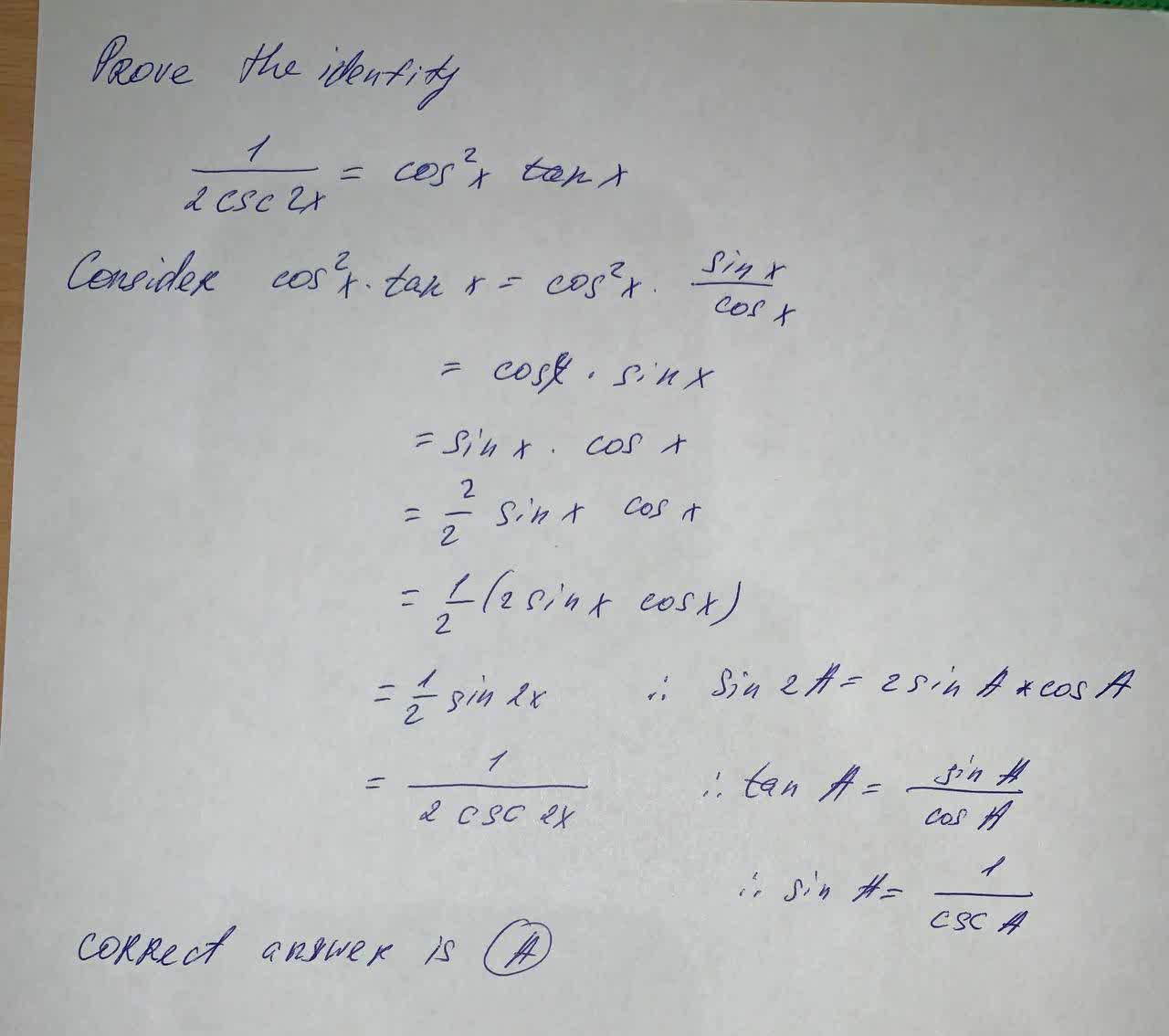#### Didn’t find what you are looking for?

Question# Prove the identity \frac{1}{2\csc 2x}=\cos^2 x \tan x Choose the sequence of steps below that verifies the identity A) \cos^2 x \tan x =\cos^2 x \frac

Trigonometric equation and identitie
ANSWEREDProve the identity
$$\frac{1}{2\csc 2x}=\cos^2 x \tan x$$
Choose the sequence of steps below that verifies the identity
A) $$\cos^2 x \tan x =\cos^2 x \frac{\sin x}{\cos x}=\cos x \sin x =\frac{\sin 2x}{2}=\frac{1}{2\csc 2x}$$
B) $$\cos^2 x \tan x=\cos^2 x \frac{\cos x}{\sin x}=\cos x \sin x=\frac{\sin 2x}{2}=\frac{1}{2 \csc 2x}$$
C) $$\cos^2 x \tan x=\cos^2 x \frac{\cos x}{\sin x}=\cos x \sin x=2 \sin 2x=\frac{1}{2 \csc 2x}$$
D) $$\cos^2 x \tan x =\cos^2 x \frac{\sin x}{\cos x}=\cos x \sin x=2 \sin 2x=\frac{1}{2 \csc 2x}$$